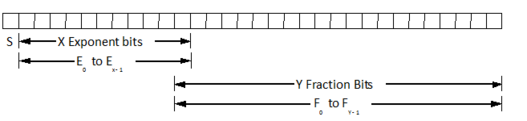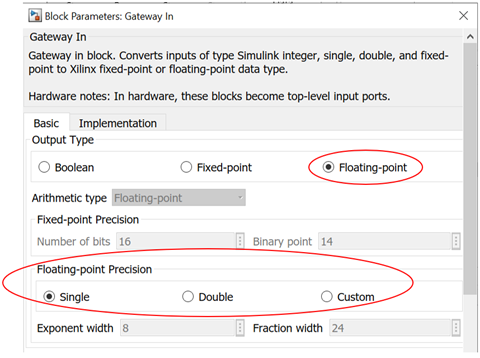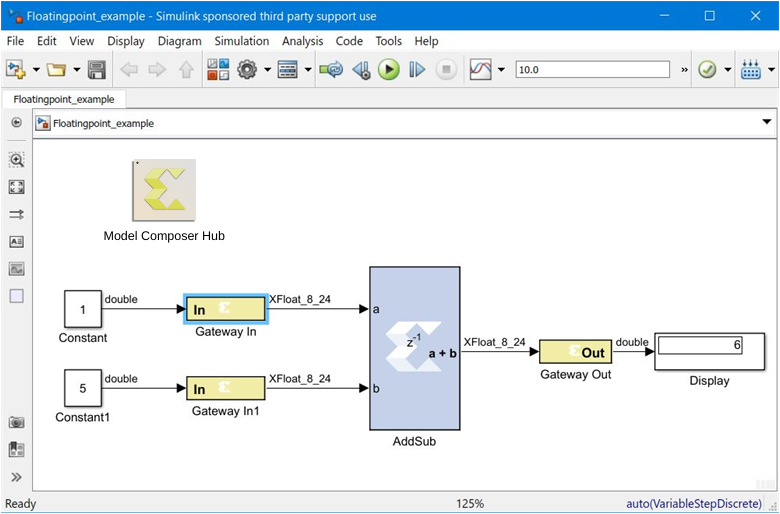# Floating-Point Data Type - 2022.1 English

## Vitis Model Composer User Guide (UG1483)

Document ID
UG1483
Release Date
2022-05-26
Version
2022.1 English

Many Model Composer HDL blocks across various libraries support the floating-point data type.

Model Composer uses the Floating-Point Operator v7.1 IP core to leverage the implementation of operations such as addition/subtraction, multiplication, comparisons and data type conversion.

The floating-point data type support is in compliance with IEEE-754 Standard for Floating-Point Arithmetic. Single precision, Double precision and Custom precision floating-point data types are supported for design input, data type display and for data rate and type propagation (RTP) across the supported HDL blocks.

## IEEE-754 Standard for Floating-Point Data Type

As shown below, floating-point data is represented using one Sign bit (S), X exponent bits and Y fraction bits. The Sign bit is always the most-significant bit (MSB).

Figure 1. Floating-Point DataAccording to the IEEE-754 standard, a floating-point value is represented and stored in the normalized form. In the normalized form the exponent value E is a biased/normalized value. The normalized exponent, E, equals the sum of the actual exponent value and the exponent bias. In the normalized form, Y-1 bits are used to store the fraction value. The F0 fraction bit is always a hidden bit and its value is assumed to be 1.

S represents the value of the sign of the number. If S is 0 then the value is a positive floating-point number; otherwise it is negative. The X bits that follow are used to store the normalized exponent value E and the last Y-1 bits are used to store the fraction/mantissa value in the normalized form.

For the given exponent width, the exponent bias is calculated using the following equation:

Exponent_bias = 2(X - 1) - 1

Where X is the exponent bit width.

According to the IEEE standard, a single precision floating-point data is represented using 32 bits. The normalized exponent and fraction/mantissa are allocated 8 and 24 bits, respectively. The exponent bias for single precision is 127. Similarly, a double precision floating-point data is represented using a total of 64 bits where the exponent bit width is 11 and the fraction bit width is 53. The exponent bias value for double precision is 1023.

The normalized floating-point number in the equation form is represented as follows:

Normalized Floating-Point Value = (-1)S x F0.F1F2 . FY-2FY-1 x (2)E

The actual value of exponent (E_actual) = E - Exponent_bias. Considering 1 as the value for the hidden bit F0 and the E_actual value, a floating-point number can be calculated as follows:

FP_Value = (-1)S x 1.F1F2 . FY-2FY-1 x (2)(E_actual)

## Floating-Point Data Representation in Model Composer

The HDL Gateway In block supports Boolean, Fixed-point, and Floating-point data types as shown in the following figure. You can select either a Single, Double or Custom precision type after specifying the floating-point data type.

For example, if Exponent width of 9 and Fraction width of 31 is specified then the floating-point data value will be stored in total 40 bits where the MSB bit will be used for sign representation, the following 9 bits will be used to store biased exponent value and the 30 LSB bits will be used to store the fractional value.

Figure 2. Floating-point PrecisionIn compliance with the IEEE-754 standard, if Single precision is selected then the total bit width is assumed to be 32; 8 bits for the exponent and 24 bits for the fraction. Similarly when Double precision is selected, the total bit width is assumed to be 64 bits; 11 bits for the exponent and 53 bits for the fraction part. When Custom precision is selected, the Exponent width and Fraction width fields are activated and you are free to specify values for these fields (8 and 24 are the default values). The total bit width for Custom precision data is the summation of the number of exponent bits and the number of fraction bits. Similar to fraction bit width for Single precision and Double precision data types the fraction bit width for Custom precision data type must include the hidden bit F0.

## Displaying the Data Type on Output Signals

As shown below, after a successful rate and type propagation, the floating-point data type is displayed on the output of each HDL block. To display the signal data type as shown in the diagram below, you select the pulldown menu item Display > Signals & Ports > Port Data Types.

Figure 3. Floating-point Data TypeA floating-point data type is displayed using the format: `XFloat_<exponent_bit_width>_<fraction_bit_width>`. Single and Double precision data types are displayed using the string "`XFloat_8_24`" and "`XFloat_11_53`", respectively.

If for a Custom precision data type the exponent bit width 9 and the fraction bit width 31 are specified, then it will be displayed as "`XFloat_9_31`". A total of 40 bits will be used to store the floating-point data value. Because floating-point data is stored in a normalized form, the fractional value will be stored in 30 bits.

In Model Composer the fixed-point data type is displayed using format `XFix_<total_data_width>_<binary_point_width>`. For example, a fixed-point data type with the data width of 40 and binary point width of 31 is displayed as `XFix_40_31`.

It is necessary to point out that in the fixed-point data type the actual number of bits used to store the fractional value is different from that used for floating-point data type. In the example above, all 31 bits are used to store the fractional bits of the fixed-point data type.

Model Composer uses the exponent bit width and the fraction bit width to configure and generate an instance of the Floating-Point Operator core.

## Rate and Type Propagation

During data rate and type propagation across a Model Composer HDL block that supports floating-point data, the following design rules are verified. The appropriate error is issued if one of the following violations is detected.

1. If a signal carrying floating-point data is connected to the port of an HDL block that doesn't support the floating-point data type.
2. If the data input (both A and B data inputs, where applicable) and the data output of an HDL block are not of the same floating-point data type. The DRC check will be made between the two inputs of a block as well as between an input and an output of the block.

If a Custom precision floating-point data type is specified, the exponent bit width and the fraction bit width of the two ports are compared to determine that they are of the same data type.

Note: The Convert and Relational blocks are excluded from this check. The Convert block supports Float-to-float data type conversion between two different floating-point data types. The Relational block output is always the Boolean data type because it gives a true or false result for a comparison operation.
3. If the data inputs are of the fixed-point data type and the data output is expected to be floating-point and vice versa.
Note: The Convert and Relational blocks are excluded from this check. The Convert block supports Fixed-to-float as well as Float-to-fixed data type conversion. The Relational block output is always the Boolean data type because it gives a true or false result for a comparison operation.
4. If Custom precision is selected for the Output Type of blocks that support the floating-point data type. For example, for blocks such as AddSub, Mult, CMult, and MUX, only Full output precision is supported if the data inputs are of the floating-point data type.
5. If the Carry In port or Carry Out port is used for the AddSub block when the operation on a floating-point data type is specified.
6. If the Floating-Point Operator IP core gives an error for DRC rules defined for the IP.